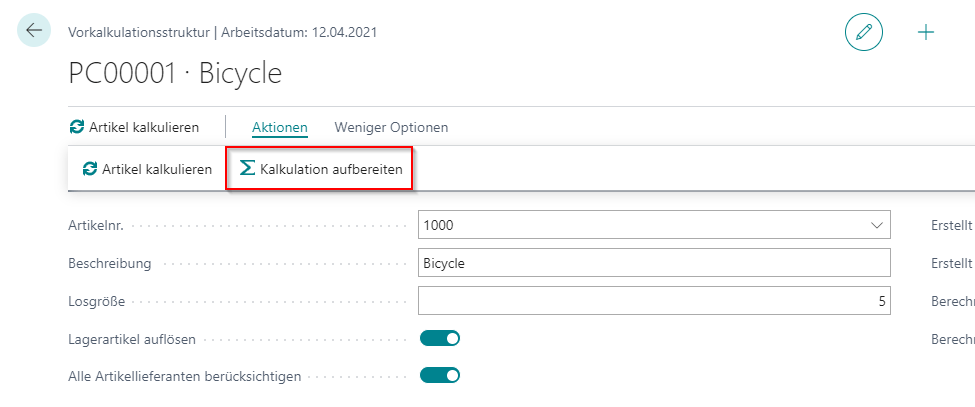1. Home
2. Docs
3. demandPlus+
4. 19*
5. Precalculation
6. Calculation

# Calculation

The calculation is the heart of the complete module. Its where boms and routings will be exploded, aswell as costs for later evalatution calculated.You can create a new calculation manually or via the calculation processes.

### Fields

For an explanation of the fields: See Fields

### Calculate item

If you want to recalculate a calculation, you can do this by clicking the “Calculate Item button.This will delete the lines and recalculate them again

### Prepare Calculation

They can prepare the calculation to apply their own schemes to the calculation.This will create a new schema for the calculation. For more information: Schemas